# sobir: Significance of Boundary Lines in R

sobir is an open-source R-library for separating illusions from boundary lines.

A modified permutation test (based on Phipson and Smyth 2010) is applied to determine whether or not there is a statistically significant constraint imposed on the maximum or minimum value of one variable relative to another. In the instances where opposite boundaries experience a constraint, this analysis is analagous to a correlation test.

The value of this approach, however, is evident in the instances when two variables aren’t correlated, but when the extremes of one are constrained at one extreme of the other. This is best illustrated using an example. A few examples are provided below.

The package has been made publically available, but is still under ongoing development. Any feedback would be appreciated.

## Installation

sobir is available on CRAN (version 0.1.0). It’s not recommended that this version be used, however, as there are some important improvements and bug fixes in version 0.1.1.9000, which can be installed using devtools.

library("devtools")
devtools::install_github("C4EcoSolutions/sobir")

## Usage

library(sobir)
library(tidyr)
library(dplyr)
library(ggplot2)

### Simulated data

#### Artificially constrained data

The data simulated below are simply two random normal variables with mean = 0, standard deviation = 1 and n = 200.

An artificial boundary effect is imposed by removing all the points beyond the line $$y = 2x + 2$$. This simulates a scenario in which the maximum value of y is constrained when $$x < 0$$.

set.seed(1)
dat_sim = tibble(x = rnorm(200, mean = 0, sd = 1),
y = rnorm(200, mean = 0, sd = 1)) %>%
mutate(bound = 2*x+2,
beyond_bound = ifelse(bound < y, TRUE, FALSE))

# Define the points that are within and beyond the artificial boundary line
dat_beyond = dplyr::filter(dat_sim, beyond_bound == TRUE)
dat_within = dplyr::filter(dat_sim, beyond_bound == FALSE)

The solid blue line is a simple linear regression line for the constrained data, indicating a poor correlation between the variables, as expected. The dashed grey line represents the artificial boundary imposed on the random data; the points that where removed are represented by the light grey open points.

We can define the area beyond the boundary as a no-data zone. Whether the labelled top-left no-data zone reflects a significant constraint on the maximum y values is to be determined.

# Visualise the simulated data with the points beyond the boundary removed
dat_within %>%
ggplot(aes(x = x, y = y)) +
geom_abline(slope = 2, intercept = 2, linetype = 2, col = "grey") +
geom_point() +
geom_point(data = dat_beyond, shape = 1, col = "lightgrey") +
geom_smooth(method = "lm", col = "blue", se = F) +
annotate(geom = "text", x = -1.5, y = 1.5, label = "no-data\nzone") +
lims(x = c(-3,3),
y = c(-3,3)) +
theme_bw()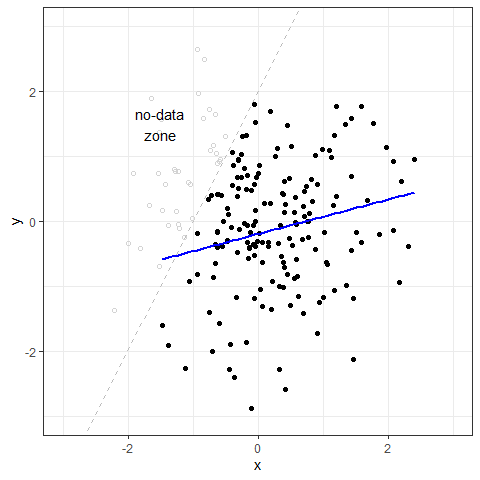To see what the package is assessing in the analysis, the boundaries and no-data zones can be extracted and visualised using the below functions.

# Extract boundary points (bpts object)
bpts_within = extract_bpts(dat_within$x, dat_within$y)

# Plot the boundaries
bpts_plot(bpts_within, xlab = "x", ylab = "y")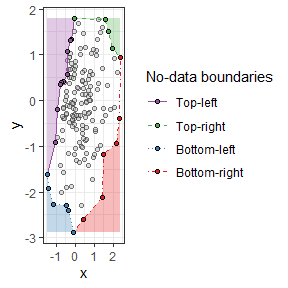The analysis involves permuting the data $$nsim$$ times to simulate a random distribution of the sample space. This is the only variable that needs to be explicitly defined. The greater $$nsim$$, the greater the precision of the analysis but the longer the analysis will take. In this instance, $$nsim = 100$$

The results can be visualised as histograms of the simulated no-data zone distributions relative to the observed no-data zone areas.

# Run the permuation test
set.seed(1)
perm_within = perm_area(dat_within$x, dat_within$y, nsim = 100, boundary = "topl")

# Plot the results
perm_plot(perm_within, histogram = T)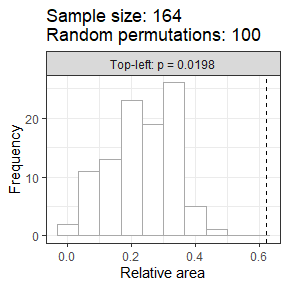As expected, the only no-data zone that shows a significant constraint is the top-left where the artificial constraint was imposed.

#### Random unconstrained data

For comparison, we can assess the significance of the same boundary on the random data without the artifical constraint to test whether or not the tool will provide a Type 1 error.

# Run the permuation test
set.seed(1)
perm_random = perm_area(dat_sim$x, dat_sim$y, nsim = 100, boundary = "topl")

# Plot the results
perm_plot(perm_random, histogram = T)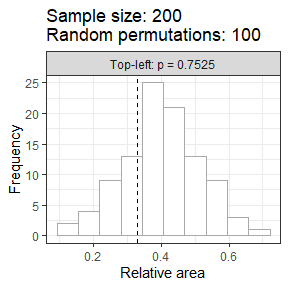As expected, the no-data zone of the random data shows no significant constraint on the top-left boundary.

### Empirical data

A similar analysis can be conducted on empirical data to test the application of the sobir methods to relationships analysed and discussed in peer-reviewed literature.

#### Sankaran et al. 2005 Determinants of Woody Cover in African Savannas

The first dataset is sourced from a Nature article on the determinants of woody cover in African savannas.

data("WoodyAfrica",package = "sobir")

One of the key figures in the article shows the woody cover from sites across the continent against the mean annual precipitation (MAP).

The data were analysed using a 99th quantile piece-wise linear regression to identify the boundary line. The gradient of a subsequent linear quantile regression for the data where MAP < 650 mm was established to be different than zero, according to the confidence intervals around the gradient.

# Extract boundary points (bpts object)
bpts_sankaran = extract_bpts(WoodyAfrica$MAP, WoodyAfrica$Cover)

# Plot the boundaries
bpts_plot(bpts_sankaran, xlab = "MAP (mm)", ylab = "Woody Cover (%)")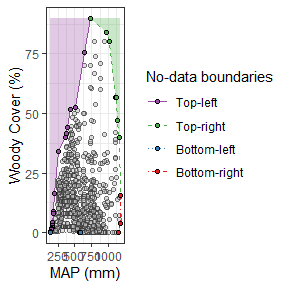The top-left boundary is hypothesised to be experiencing a significant constraint, which reflects a constraint of the maximum woody cover at low mean annual precipitation.

# Run the permuation test
set.seed(1)
perm_sankaran = perm_area(WoodyAfrica$MAP, WoodyAfrica$Cover, nsim = 100, boundary = "topl")

# Plot the results
perm_plot(perm_sankaran, histogram = T)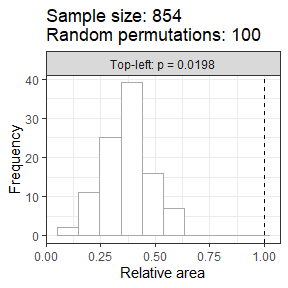The null hypothesis that the top-left boundary is random can be rejected as the p-value is less than 0.05.

#### Mills et al. 2017 Effects of anabolic and catabolic nutrients on woody plant encroachment after long-term experimental fertilization in a South African savanna

The second empirical dataset is sourced from a PLoS ONE article on the effects of different soil nutrients on woody plant encroachment after long-term experimental fertilization.

data("WoodyTowoomba", package = "sobir")

One of the key figures in the article shows the number of trees against the ratio of soil Mn/Cu.

The data were statistically analysed by aggregating the tree abundance into four bins (0-1, 2-4, 5-8, >8) and running a Kruskal-Wallis rank sum test on soil Mn/Cu across the bins. The soil Mn/Cu ratios were shown to be significantly greater where tree abundance was greater (more individual trees per plot).

WoodyTowoomba = WoodyTowoomba %>%
mutate(MnCu = Mn/Cu)

# Extract boundary points (bpts object)
bpts_mills_mncu = extract_bpts(WoodyTowoomba$MnCu, WoodyTowoomba$TreeNum)

# Plot the boundaries
bpts_plot(bpts_mills_mncu, xlab = "Soil Mn/Cu", ylab = "Tree abundance")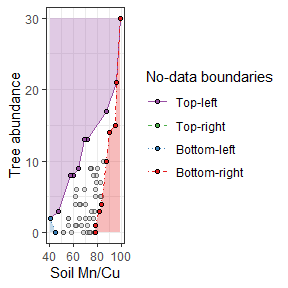In accordance with the Catabolic Theory, it was inferred that there were boundary effects occuring at both the top-left and bottom-right boundaries that represent a constraint on the maximum tree abundance at lower Mn/Cu ratios and a constraint on the minimum tree abundance at greater Mn/Cu ratios.

# Run the permuation tests
set.seed(1)
perm_mills_topl = perm_area(WoodyTowoomba$MnCu, WoodyTowoomba$TreeNum, nsim = 100, boundary = "topl")
perm_mills_botr = perm_area(WoodyTowoomba$MnCu, WoodyTowoomba$TreeNum, nsim = 100, boundary = "botr")

# Plot the results
perm_plot(perm_mills_topl, histogram = T)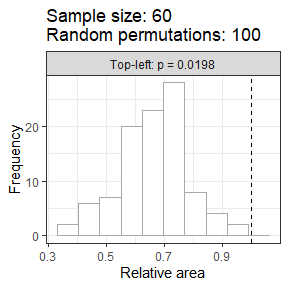perm_plot(perm_mills_botr, histogram = T)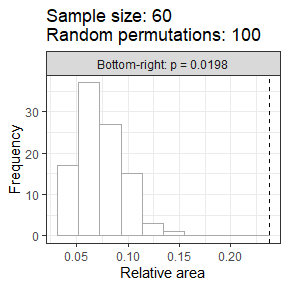The null hypotheses that the top-left and bottom-right boundaries are random can both be rejected as the p-values are both less than 0.05.

## Vignettes

sobir: brief explainer